# Polynomial

(diff) ← Older revision | Latest revision (diff) | Newer revision → (diff)

An expression of the form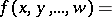where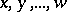are variables and(the coefficients of the polynomial) and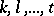(the exponents of the powers, which are non-negative integers) are constants. The individual expressions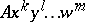are called the terms of the polynomial. The order of the terms, and also the order of the factors in each term, can be changed arbitrarily; in precisely the same way it is possible to introduce or omit terms with zero coefficients and, in each individual term, zero powers. When the polynomial has one, two or three terms it is called a monomial, binomial or trinomial.

With regard to the coefficients of a polynomial one assumes that they belong to a field, for example, the field of rational, real or complex numbers.

Two terms of a polynomial are called similar if the powers of the same variables in them are equal. Terms similar to each other,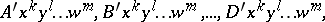can be replaced by one term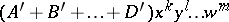(reduction of similar terms). Two polynomials are called equal if, after reduction, all terms with non-zero coefficients are pairwise identical (but, possibly, written in a different order), and also if all the coefficients of both of these polynomials turn out to be zero. In the latter case the polynomial is called identically zero and is denoted by the symbol 0.

The sum of the powers of any term of a polynomial is called the degree of that term. If the polynomial is not identically zero, then among the terms with non-zero coefficients (it is assumed that similar terms have been reduced) there is at least one of highest degree: this highest degree is called the degree of the polynomial. The zero polynomial does not have a degree. A polynomial of degree zero reduces to a single term(a constant, not equal to zero).

A polynomial in the variablesis called a symmetric polynomial if it is not changed by any permutation of the variables. A polynomial of which all terms have the same degree is called a homogeneous polynomial or a form; forms of the first, second or third degree are called linear, quadratic or cubic, and, according to the number of variables (two or three), they are called dyadic (binary) or triadic (ternary) (for example,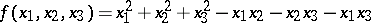is a ternary quadratic form).

The degree of a polynomial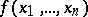with respect to one of its variables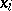,, is the highest power with whichoccurs in a term of this polynomial (this degree may be zero). Of two terms of a polynomial the higher one (relative to a given numbering of the variables) is that for which the power of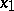is higher, and if these powers are equal, that for which the power of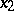is higher, etc. If all terms of a polynomial are ordered so that each term is lower than the preceding, then the terms are said to be lexicographically ordered. The term which then stands in the first place is called the highest term (or leading term). A polynomial of one variable with lexicographically ordered terms has the formwhereare the coefficients.

The roots of a polynomial in one variable over a fieldare the solutions of the algebraic equation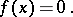The roots of a polynomial are related to its coefficients by Viète's formula (see Viète theorem).

The set of all possible polynomials invariables with coefficients from a given field forms a ring with respect to the naturally defined operations of addition and multiplication. The ring of polynomials in an infinite set of variables can also be considered. A ring of polynomials is an associative-commutative ring without zero divisors (that is, a product of non-zero polynomials cannot be 0).

If for two given polynomialsandthere exists a polynomialsuch that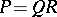, then one says thatis divisible by;is called the divisor andthe quotient. Ifis not divisible by, but both polynomials contain the same variable, for example, and the degree ofrelative toisand the degree ofrelative tois,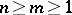, then there are polynomials,andsuch that, wheredoes not containat all andoccurs inwith degree less than. Whenis the only variable, thencan be taken to be 1; in this case the operation of findingandfromandis called division with remainder; division with remainder can be carried out using the Horner scheme.

By repeated application of this operation it is possible to find the greatest common divisor ofand, that is, the divisor ofandwhich is divisible by any common divisor of these polynomials (see Euclidean algorithm). Two polynomials with greatest common divisor equal to 1 are called coprime.

A polynomial which can be represented as a product of polynomials of smaller degree with coefficients from a given field is called reducible (over that field); otherwise it is called irreducible. The irreducible polynomials play a role in the ring of polynomials similar to that played by the prime numbers in the ring of integers. For example, the following theorem holds: If a productis divisible by an irreducible polynomialandis not divisible by, thenmust be divisible by. Each polynomial of degree greater than zero splits over a given field into a product of irreducible factors in a unique way (up to factors of degree zero). For example, the polynomial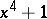is irreducible over the field of rational numbers, splits into two factors over the field of real numbers and into four factors over the field of complex numbers. In general, each polynomial of one variablewith real coefficients splits over the field of real numbers into factors of the first and second degree, and over the field of complex numbers into factors of the first degree (cf. Algebra, fundamental theorem of). For two or more variables this is no longer true. Over any field, forthere are polynomials invariables that are irreducible over any extension of. Such polynomials are called absolutely irreducible. For example, the polynomial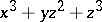is irreducible over any number field.

If the variablesare given numerical values (for example, real or complex), then the polynomial assumes a certain numerical value. Thus, a polynomial can be considered as a function of the corresponding variables. This function is continuous and differentiable for any values of the variables; it can be characterized as an entire rational function, that is, a function obtained from variables and constants (the coefficients) by performing in a specific order the operations of addition, subtraction and multiplication. Entire rational functions belong to the broader class of rational functions, where division is added to the list of operations: Any rational function can be represented as a quotient of two polynomials. Finally, rational functions are contained in the class of algebraic functions (cf. Algebraic function).

One of the most important properties of polynomials is that any continuous function on a compact subset of the complex plane can be approximated by a polynomial within arbitrarily small error (see Weierstrass theorem).

Special systems of polynomials, orthogonal polynomials, are used in approximation theory as a means of representing functions by series.